# Motor Efficiency Formula

Today, it has become much easier for engineers and manufacturers to design (or specify) electric motors that work. However, a challenge that has remained constant is the need to design (or specify) an electric motor that converts electrical energy into mechanical energy with little to no losses.

One of the major parameters that allow engineers to evaluate the performance of an electric motor is motor efficiency. Motor efficiency simply describes how well the motor converts electrical energy into mechanical energy. A highly efficient motor converts a large proportion of the electrical energy into mechanical energy, allowing low operating costs, smooth speed and operation over a required range, and little to no vibrations.

This article presents helpful information about motor efficiency and performance. It will also cover the different types of losses that typically affect the performance of electric motors.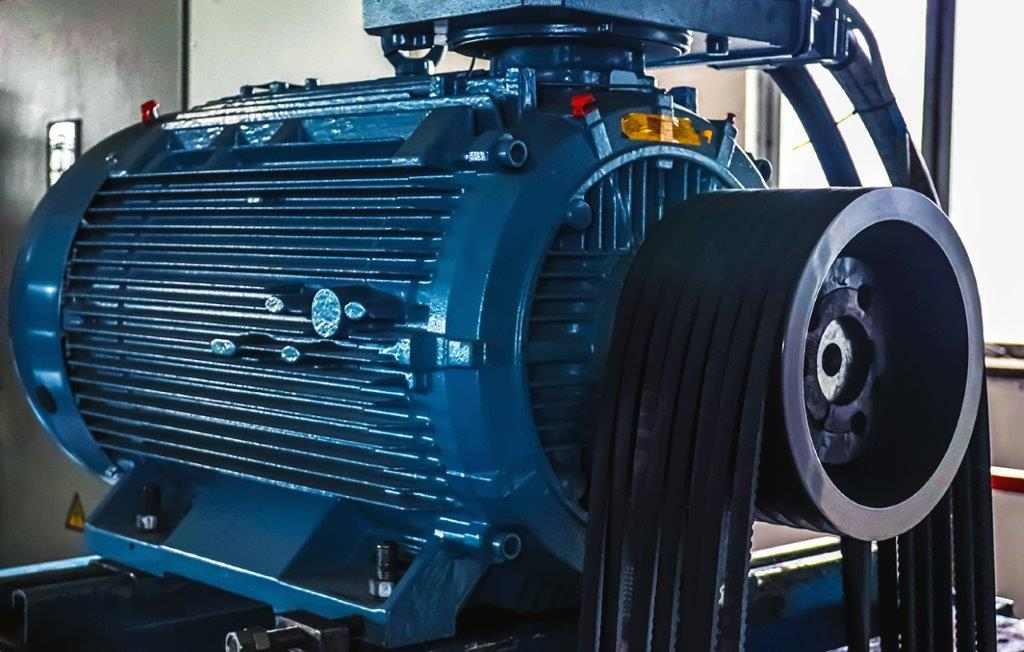Back to the basics in electric motor performance and key formula for motor efficiency

## The formula for motor efficiency

The efficiency of a motor is simply the ratio of mechanical power output to the electric power input to the motor. The following subsections will provide formulas for estimating motor efficiency when the power output is presented in watt and horsepower.

## The formula for motor efficiency when the power output is measured in watt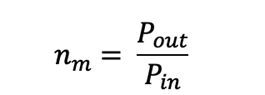Where:

nm=motor efficiency

Pout=Shaft power output (watt)

Pin=Electric power input (watt)

## The formula for motor efficiency when the power output is measured in horsepower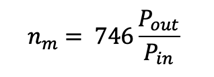Where:

nm=motor efficiency

Pout=Shaft power output (horsepower, hp)

Pin=Electric power input (watt)

100% efficiency means that the electric motor converts all of the electrical energy into useful work. However, this is impossible to achieve; electric motors experience several types of losses during operation, causing them to have less than 100% efficiency. Engineers will have to consider several factors and design considerations to create a motor with an efficiency that is close to being perfect.

## Electric motor efficiency losses: here is what every engineer must know

There are four types of losses engineers should look out for in electric motors. They include:

1. Ohmic losses
2. Core losses
3. Mechanical losses
4. Stray losses

### No.1: Ohmic losses

Ohmic losses describe the losses that occur due to electric current flowing through the conductors of the motor. These losses typically occur in the primary and secondary windings of the motor in the form of heat and can be calculated as follows.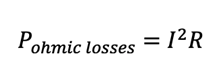Where:

R=Electrical resistance (Ohms)

I=Current (A)

When designing or specifying conductors for electric motors, it is always recommended to opt for copper coils rather than aluminum coils. This is because copper conductors generally have higher conductivity (and lower resistivity or electrical resistance) than aluminum conductors. So, an aluminum conductor will have higher ohmic losses than a similarly shaped copper conductor for the same electric current.

In addition, engineers can reduce ohmic losses by using a thicker gauge copper wire and improving manufacturing techniques to shorten the end windings.

### No.2: Core losses

Core losses, also called iron losses, are associated losses in the magnetic paths of the motor. They can be further divided into:

1. Hysteresis losses
2. Eddy current losses.

Hysteresis losses result from the reorientation of the magnetic field within the motor’s lamination. They are affected by the total flux density and core material’s ability to change polarity easily.

In contrast, eddy current losses result from electrical currents produced between laminations due to changing magnetic fields. They are affected by the total flux density, the frequency at which the polarity of the flux changes, and the thickness of the part’s lamination.

As a rule, it is recommended to use thin laminations made of higher-grade steel to reduce hysteresis and eddy current losses.

### No.3: Mechanical losses

Mechanical losses result from friction in the motor bearings or bushings in brushed type DC motors. Engineers can reduce motor bearing friction and prevent wear by properly lubricating the motor bearing with grease.

### No.4: Stray losses

Stray losses are minor losses that typically remain after ohmic losses, core losses, and mechanical losses.

## Motor amperage calculation and electric motor performance

Motor amperage (also called the full load amp) is the largest current that the motor is designed to carry under particular conditions. The following sections present formulas to calculate full load amps (FLA) of single-phase and three-phase circuits.

## Single-phase motor FLA calculator

When the motor power rating (Pout) is specified in kilowatts (kW), the single-phase motor FLA can be calculated using: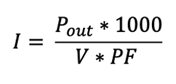In contrast, when the output power (Pout) is specified in horsepower (hp), the single-phase motor FLA can be calculated using: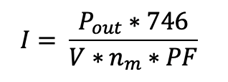Where:

Pout=mechanical power output

PF=Power factor

V=Line voltage

nm=The efficiency of the motor

## Three-phase motor full load amps calculator

When the motor power rating (Pout) is specified in kilowatt (kW), the three-phase motor FLA can be calculated using: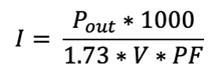In contrast, when the output power (Pout) is specified in horsepower (hp), the three-phase motor FLA can be calculated using: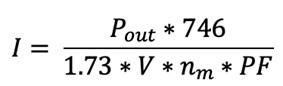Where:

Pout=mechanical power output

PF=Power factor

V=Line voltage

nm=The efficiency of the motor

Engineers should ensure that the input current to the motor is less than the full load amps. Subjecting a motor to input currents higher than the motor FLA typically causes overheating, which in turn damages the motor's windings and insulation and affects motor performance.

## Conclusion

While this article presents helpful information about electric motor efficiency, performance, and full load amperage, there are still several other factors that influence the performance of electric motors. For example, thermal design, assembly techniques, and aerodynamics are vital to the performance of electric motors. Engineers are advised to reach out to motor manufacturers to discuss their application requirements.